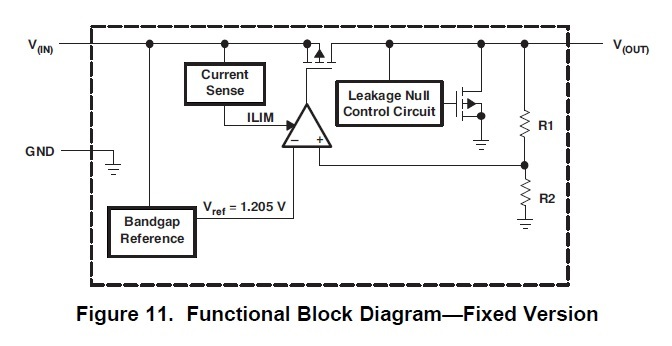If you have a related question, please click the "Ask a related question" button in the top right corner. The newly created question will be automatically linked to this question.

# TPS715: TPS715, Fixed version, Internal divided resister

Part Number: TPS715

Our customer ask me about the value of the internal divided resister(R1 and R2) in TPS71525.Regards,

Tamio

• Hi Tamio,

Please allow a day or two for us to lookup this information. Please keep in mind that the ratio is trimmed and ensured at final test rather than the exact resistance value.

Very Respectfully,
Ryan
• Hi Tamio,

The internal reference is 1.205 V and the internal R2 is nominally 843 kΩ. As a result R1 is approximately 906 kΩ for the 2.5 V version of TPS715. Please keep in mind that the ratio is trimmed and ensured at final test rather than the exact resistance value.

Very Respectfully,
Ryan
• Hi, Ryan

Than you for sharing your information.
It is helpful for our customer to design his circuit.

If you have the evaluation board which TPS71530 was mounted, please confirm the discharging time until Vo=2.0V from Vo=3.0V.
If possible, the output capacitor is total 100uF. His target time is 10sec.

Thanks
Tamio
• Hi Tamio,

The discharge of the capacitor can be calculated but it is important to keep in mind that the internal resistor divider is only one discharge path. If the load is still actively drawing current, the discharge time will change.

Vc = V0 x e^(-t / (R x C))

Vc is the voltage across the capacitor
V0 is the initial capacitor voltage
t is time
R is the internal resistor divider
C is the capacitance

Assuming that the only discharge path is the internal resistor divider:

2 V = 3 V x e^(-t / ((843 k + 906 k) x 100 uF))
2 V / 3 V = e^(-t / (1.749 M x 100 uF))
2 / 3 = e^(-t / 174.9)
ln(2/3) = -t / 174.9
t = 70.92 s

Without another discharge path, the capacitor will not be discharged in 10 s.

Very Respectfully,
Ryan